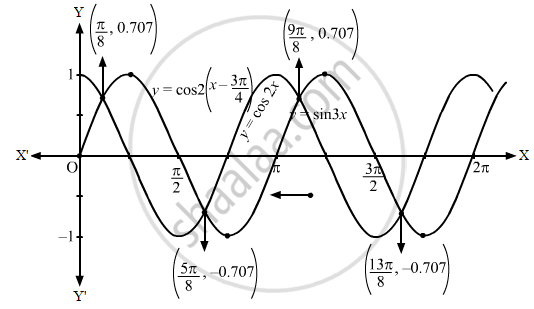Advertisement Remove all ads

# Sketch the Graph of the Following Curves on the Same Scale and the Same Axes: Y = Cos 2 X and Y = Cos 2 ( X − π 4 ) - Mathematics

Answer in Brief

Sketch the graph of the following curves on the same scale and the same axes:
$y = \cos 2x\text{ and }y = \cos 2\left( x - \frac{\pi}{4} \right)$

Advertisement Remove all ads

#### Solution

First, we draw the graph of y = cos 2x.
Let us now draw the graph of $y = \cos2\left( x - \frac{\pi}{4} \right)$

$y = \cos2\left( x - \frac{\pi}{4} \right)$

$\Rightarrow y - 0 = \cos2\left( x - \frac{\pi}{4} \right) . . . (i)$

$\text{ On shifting the origin at }\left( \frac{\pi}{4}, 0 \right), \text{ we get: }$

$x = X + \frac{\pi}{4}\text{ and }y = Y + 0$

On subsitituting the values in (i), we get:

$Y = \cos2X$

$\text{ Then, we draw the graph of Y }= \cos2X\text{ and shift it by }\frac{\pi}{4}\text{ to the right.}$

Then, we will obtain the following graph:Concept: Graphs of Trigonometric Functions
Is there an error in this question or solution?
Advertisement Remove all ads

#### APPEARS IN

RD Sharma Class 11 Mathematics Textbook
Chapter 6 Graphs of Trigonometric Functions
Exercise 6.2 | Q 2.2 | Page 8
Advertisement Remove all ads
Advertisement Remove all ads
Share
Notifications

View all notifications

Forgot password?